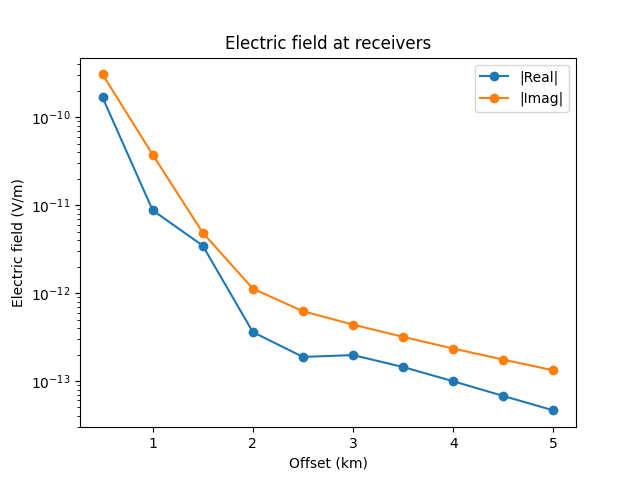Getting started¶

The main modelling routine is empymod.model.bipole, which can compute the electromagnetic frequency- or time-domain field due to arbitrary finite electric or magnetic bipole sources, measured by arbitrary finite electric or magnetic bipole receivers. The model is defined by horizontal resistivity and anisotropy, horizontal and vertical electric permittivities and horizontal and vertical magnetic permeabilities. By default, the electromagnetic response is normalized to source and receiver of 1 m length, and source strength of 1 A.

Basic example¶

A simple frequency-domain example, where we keep most of the parameters left at the default value:

In : import empymod
...: import numpy as np
...: import matplotlib.pyplot as plt
...:

First we define the survey parameters: source and receiver locations, and source frequencies.

In : # x-directed bipole source: x0, x1, y0, y1, z0, z1
...: source = [-50, 50, 0, 0, -100, -100]
...: # Source frequency
...: frequency = 1
...: offsets = np.arange(1, 11)*500
...: # x-directed dipole receiver-array: x, y, z, azimuth, dip
...: receivers = [offsets, offsets*0, -200, 0, 0]
...:

Next, we define the resistivity model:

In : # Layer boundaries
...: depth = [0, -300, -1000, -1050]
...: # Layer resistivities
...: resistivities = [1e20, 0.3, 1, 50, 1]
...:

And finally we compute the electromagnetic response at receiver locations:

In : efield = empymod.bipole(
...:         src=source,
...:         depth=depth,
...:         res=resistivities,
...:         freqtime=frequency,
...:         verb=4,
...: )
...:

:: empymod START  ::  v2.1.3

depth       [m] :  -1050 -1000 -300 0
res     [Ohm.m] :  1 50 1 0.3 1E+20
aniso       [-] :  1 1 1 1 1
epermH      [-] :  1 1 1 1 1
epermV      [-] :  1 1 1 1 1
mpermH      [-] :  1 1 1 1 1
mpermV      [-] :  1 1 1 1 1
direct field    :  Comp. in wavenumber domain
frequency  [Hz] :  1
Hankel          :  DLF (Fast Hankel Transform)
> Filter      :  Key 201 (2009)
> DLF type    :  Standard
Loop over       :  None (all vectorized)
Source(s)       :  1 bipole(s)
> intpts      :  1 (as dipole)
> length  [m] :  100
> strength[A] :  0
> x_c     [m] :  0
> y_c     [m] :  0
> z_c     [m] :  -100
> azimuth [°] :  0
> dip     [°] :  0
> x       [m] :  500 - 5000 : 10  [min-max; #]
:  500 1000 1500 2000 2500 3000 3500 4000 4500 5000
> y       [m] :  0 - 0 : 10  [min-max; #]
:  0 0 0 0 0 0 0 0 0 0
> z       [m] :  -200
> azimuth [°] :  0
> dip     [°] :  0
Required ab's   :  11

:: empymod END; runtime = 0:00:00.005233 :: 1 kernel call(s)

Let’s plot the resulting responses:

In : fig, ax = plt.subplots()
...: ax.semilogy(offsets/1e3, abs(efield.real), 'C0o-', label='|Real|')
...: ax.semilogy(offsets/1e3, abs(efield.imag), 'C1o-', label='|Imag|')
...: ax.legend()
...: ax.set_xlabel('Offset (km)');
...: ax.set_ylabel('Electric field (V/m)');
...:A good starting point is the Gallery-gallery or [Wert17b], and more detailed information can be found in [Wert17]. The description of all parameters can be found in the API documentation for empymod.model.bipole.

Structure¶

The structure of empymod is:

• model.py: EM modelling; principal end-user facing routines.

• utils.py: Utilities such as checking input parameters.

• kernel.py: Kernel of empymod, computes the wavenumber-domain electromagnetic response. Plus analytical, frequency-domain full- and half-space solutions.

• transform.py: Methods to carry out the required Hankel transform from wavenumber to space domain and Fourier transform from frequency to time domain.

• filters.py: Filters for the Digital Linear Filters method DLF (Hankel and Fourier transforms).

Coordinate system¶

The used coordinate system is either a

• Left-Handed System (LHS), where Easting is the $$x$$-direction, Northing the $$y$$-direction, and positive $$z$$ is pointing downwards;

• Right-Handed System (RHS), where Easting is the $$x$$-direction, Northing the $$y$$-direction, and positive $$z$$ is pointing upwards.

Have a look at the example Definition of the coordinate system in empymod for further explanations.

Theory¶

The code is principally based on

• [HuTS15] for the wavenumber-domain computation (kernel),

• [Key12] for the DLF and QWE transforms,

• [SlHM10] for the analytical half-space solutions, and

• [Hami00] for the FFTLog.

See these publications and all the others given in the References, if you are interested in the theory on which empymod is based. Another good reference is [ZiSl19]. The book derives in great detail the equations for layered-Earth CSEM modelling.